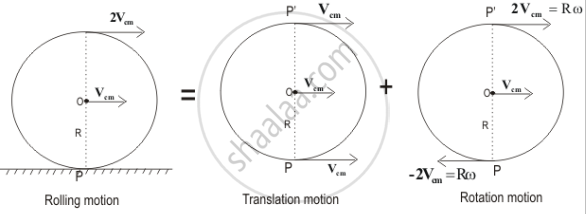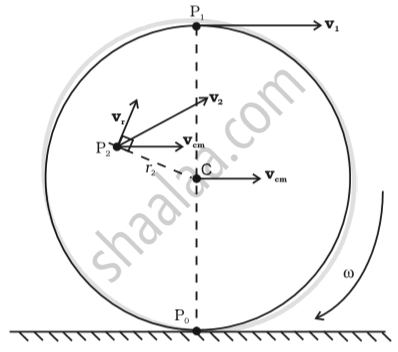# Rolling Motion

#### notes

Rolling motion

Rolling motion is a combination of rotation and translation.All the particles on a rolling body have two kinds of velocity

1. Translational, which is velocity of COM.

2. Linear velocity on account of rotational motion.Here in the figure we can see that every point have two velocities, one in the direction of velocity of COM and other perpendicular to the line joining centre and the point.

• Point Po have opposite velocities , and if condition of no-slipping is there then it must have zero velocity, so V_(com) = ωR

• At point P1both the velocities add up.

• At any other point, add both the velocities vectorially to get the resultant, which are shown for some of the cases in red color in figure.

• The line passing through PO and parallel to w is called the instantaneous axis of rotation.

• The point PO is instantaneously at rest.

• Kinetic Energy of Rolling Motion

• "KE_rolling" = "KE_translation" + "KE_rotation"

• KE=1/2Iomega^2 + 1/2mv_(com)^2
• substituting I =mk2(where K is the radius of gyration) and V_"com"=Romega
• we get "KE"=1/2mv_"com"^2(1+k^2/R^2)

#### description

• Linear Acceleration and Speed While Pure Rolling Down an Inclined Plane
If you would like to contribute notes or other learning material, please submit them using the button below.

### Shaalaa.com

Combined Translation + Rotational Motion [00:55:54]
S
0%